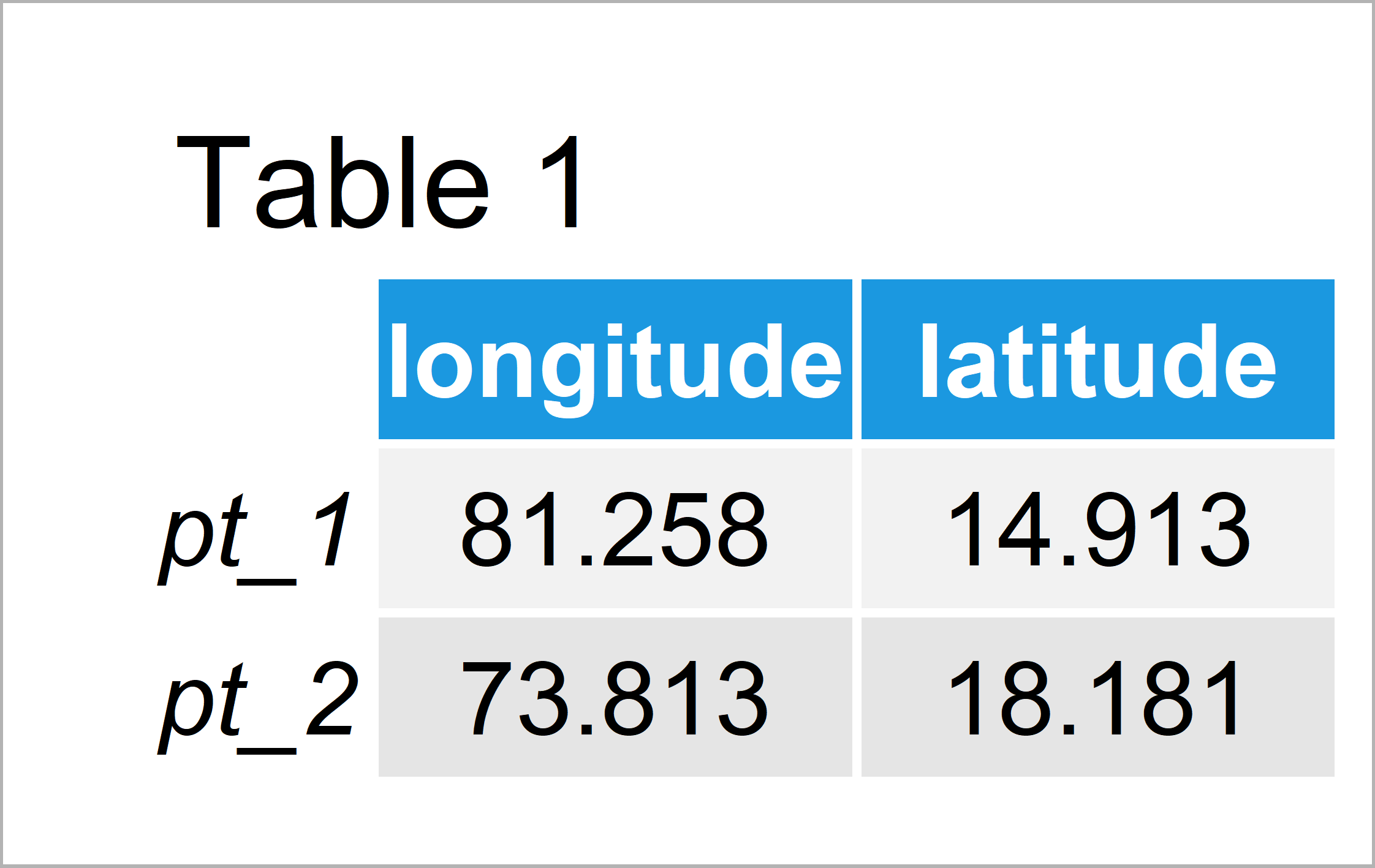# Law of Cosines Great Circle Distance in R (Example)

In this R tutorial you’ll learn how to calculate the Law of Cosines distance.

The content looks as follows:

It’s time to dive into the programming part:

## Exemplifying Data

We’ll use the following data as basement for this R tutorial:

```my_points <- matrix(c(81.25798, 73.81277,    # Create longitude/latitude matrix
14.91254, 18.18145),
nrow = 2)
colnames(my_points) <- c("longitude", "latitude")
rownames(my_points) <- c("pt_1", "pt_2")
my_points                                    # Print longitude/latitude matrix```Table 1 shows that the example data is composed of two longitude and latitude points.

## Example: Calculate Law of Cosines Great Circle Distance Using distCosine() Function of geosphere Package

The following R programming syntax illustrates how to apply the distCosine function of the geosphere package to calculate the Law of Cosines great circle distance in R.

First, we need to install and load the geosphere package:

```install.packages("geosphere")                # Install geosphere package

Now, we can use the distCosine function to return the distance between our two geospatial points:

```my_dist <- distCosine(my_points)             # Calculate Law of Cosines distance
my_dist                                      # Print Law of Cosines distance
#  873680.6```

The geographical distance between our two data points according to the Law of Cosines is 873680.6.

## Video, Further Resources & Summary

Have a look at the following video of the Statistics Globe YouTube channel. In the video, I’m explaining the R programming codes of this post in RStudio:

Furthermore, you may want to read the related R articles which I have published on https://statisticsglobe.com/:

In summary: This article has illustrated how to compute the geospatial Law of Cosines in the R programming language. Don’t hesitate to tell me about it in the comments, in case you have additional questions.

Subscribe to the Statistics Globe Newsletter